## Cafeteria UML Deployment

17
0
3
publish time: 2021-03-30Kiraaaa

The UML Collaboration Diagram is used to show the relationship between the objects in a system. Instead of showing the flow of messages, a collaboration diagram depicts the architecture of the object residing in the system as it is based on object-oriented programming. The UML Collaboration diagrams are used by designers to define and clarify the roles of the objects that perform a particular flow of events of a use case. The UML Collaboration diagram is the primary source of information used to determine class responsibilities and interfaces. UML Collaboration Diagrams tend to be better suited to depict simpler interactions of smaller numbers of objects. To understand UML Collaboration Diagram, let’s have a look at the following UML collaboration diagram built using EdrawMax. It shows the Cafeteria UML Collaboration and how a customer visits the cafe and eats the desired food and pays the requested sum.

See More Related TemplatesThis is an example of a UML diagram about adding events to the calendar.
UML Diagram - Add event to calendar
23
1
0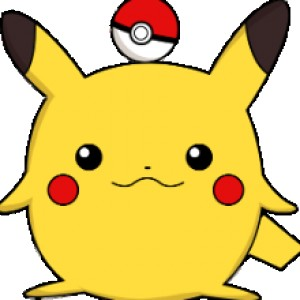easy diagrams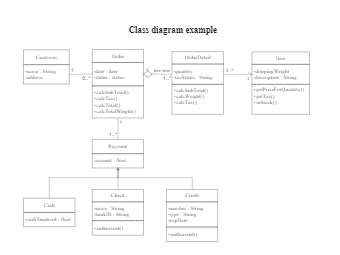This is an example of a uml class diagram.
Class diagram example
187
5
4easy diagrams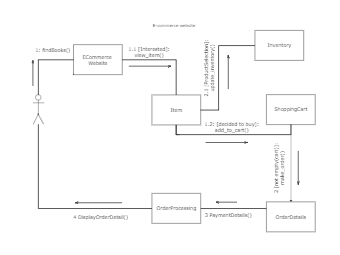This is an example of a UML collaboration diagram.
ecommerce website - UML diagram
20
2
0easy diagrams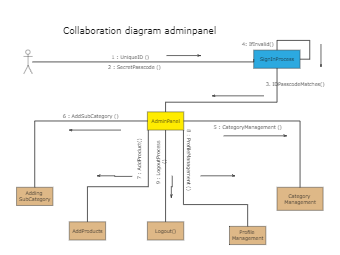10
1
0easy diagrams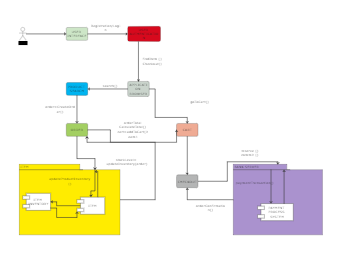This is an example of a UML communication diagram.
Communication diagram example
11
1
1easy diagrams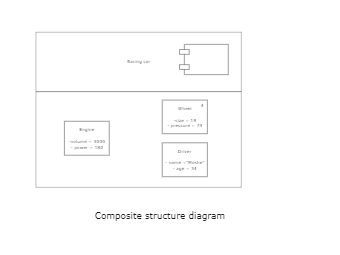This is an example of a composite structure diagram.
Composite structure diagram
3
0
0easy diagrams